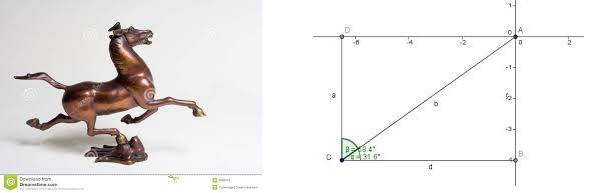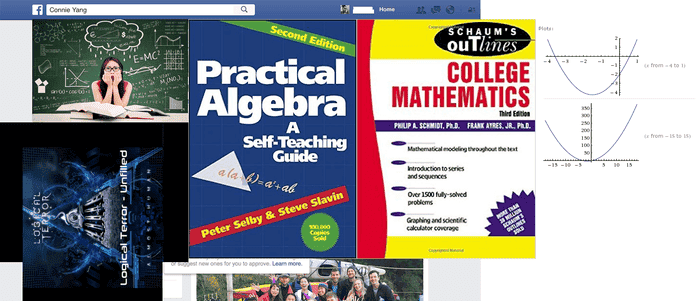I have a few doubts about graphs ...
and how variables of an equation or expression are going to look like on a graph .... ?

are they only going to look like single points in graph ??

when we deal with the variable x .. do we deal it as an object ??

I mean .. most of the basic questions are like .. an object is moving through a space with velocity ... and its points in graph is varying at different points ...

find or try to understand the velocity of the object x .. at a specific point ?

what if the object is a bit large ... ?

what I have in my mind sort of looks like these ...

I was wondering how graphs of these things are plotted ?? do they make multiple points in the graph of the path of their motion ??? as in not simply a point ??are they going to look like a messed up graph like this one ???if so , which equations do i associate these advanced graphs with ???

Last edited by a moderator:

pbuk
Gold Member
You should learn more about what mathematicians mean by a graph (of a function); if you have not learned any of this at school you could try Khan Academy (you might like to start by revising algebra basics to make sure you understand the explanations that are used later).

When we plot the motion of a solid object we usually represent the object by a single point at its centre of mass - we say that we represent the object as a "point mass".

that is a very new information to me ... i have never heard anyone talking about a "point mass " when doing maths ...

i have lot more questions to ask ... not sure where to begin and where to end .. right now ..

i have been trying to stare into things like these for some time now ...just trying to understand some college level maths in terms of graphs ...

is there such a thing called regions in graph ? or are they only represented by points ??
does these regions have some sort of name ???

few more questions about graphing ... i was not familiar with this "point mass " concept ... until now ...

this concept of point mass makes graphing a little more easier .. yea ?

every time i try to plot an expression or equation ... i look it as a "point mass " ???

for example .. that function ...

actually i was lost between a lot of stuffs until now ...

from algebraic expressions to equations ...graphs ... to the roots of functions ...

i am trying to imagine a function for that electro magnetic small train ....

my first question would be what does it mean by finding the roots of such an equation ...?

the definition of a function is like a solution that makes the whole equation zero or close to zero ??

am i trying to find the root of such an equation ?

what good is it to find the solution of that function which makes the graph of the small electro magnetic train zero ??

should i be looking for something else ?

it could be like i am confusing .. finding roots .. with differentiation or integration ... ???

pbuk
Gold Member
I think you need to go and learn the basics of these subjects before you ask random questions, many of which don't make sense. Even for the ones that do make sense, if you haven't learned the basics you won't understand the answers.

DrClaude
Mentor
I'm sorry, but this is a mishmash of questions that are not completely coherent. And the pictures you are posting are not helping.

•